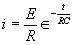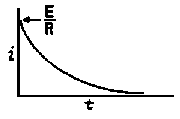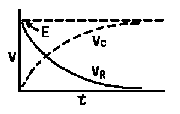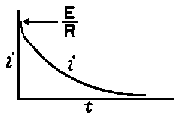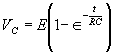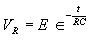## Transient I and E in LCR Circuits

 The formulas whhich follow may be used to closely  approximate the growth and decay of current and   voltage in circuits involving L, C and R      where    i  =  instantaneous current in amperes at any                      given time (t),               E  =  potential in volts as designated,               R  =  circuit resistance in ohms,               C  =  capacitance in farads,               L  =  inductance in henrys,               V  =  steady state potential in volts,              VC =   reactive volts across C,              VL =   reactive volts across L,              VR =   voltage across R, RC  =  time constant of RC circuit in seconds,          L/R  =  time constant of RL circuit in seconds,             t   =  any given time in seconds after switch                     is thrown,             e   =  a constant, 2.718 (base of the natural                      system of logarithms),          Sw  =  switch     The time constant is defined as the time in seconds  for current or voltage to fall to 1/e or 36.8% of its initial  value or to rise to (1 - 1/e) or approximately 63.2%  of its final value. Charging a de-energized Capacitive Circuit Discharging an Energized Capacitive Circuit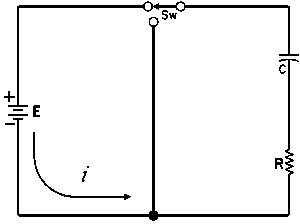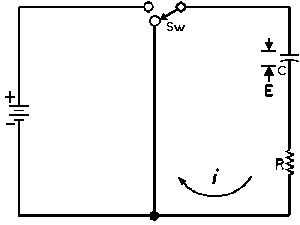E  =  applied potential. E  =  potential to which C ischarged prior to closing Sw.